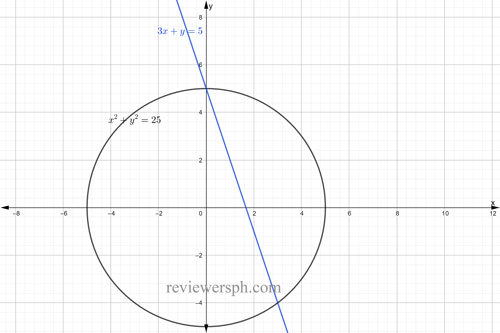### Math Notes

Subjects

#### Analytic Geometry Solutions

##### Topics || Problems

Find the point of intersection of the curve $$x^2 +y^2 = 25$$ and $$3x + y =5$$. Solution:

Graph the equations to estimate the intersection.Isolate $$y$$ from $$3x+y = 5$$

$$y = 5-3x$$

Substitute this value to the equation of the circle to solve $$x$$

$$x^2 +(5-3x)^2 = 25$$

$$x^2 + 25 -30x +9x^2 = 25$$

$$x^2 -30x +9x^2 =0$$

$$10x^2 -30x = 0$$

$$x = 3$$ and $$x = 0$$

$$y = 5-3(0) = 5$$

$$y = 5-3(3) = -4$$

The point of intersections are @ $$(0,5)$$ and (3,-4)